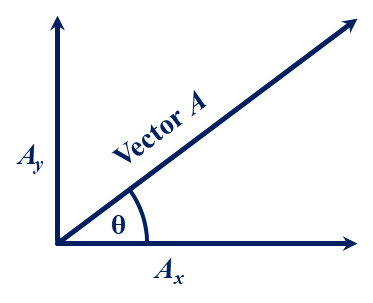﻿ Week 6 Doc O's FHS Physics Assignment Page

Week 6:  12 October to 16 October 2020

11 October 2020:

No Class:  Columbus Day holiday.

12 October 2019:

In Class:  Motion in two dimensions.

Expectations:  Students will be able to solve problems with motion in two dimensions using vertical components.

Due:  Pages 1:152-153 (126 – 134).

Homework:  Pages 1:153-156 (135 – 153).

13 October 2020:

In Class:  Projectiles Fired at an Angle.

• Ay = A sin θ
Ax = A cos θ
• A = Any vector
Ay = Vertical component of A
Ax = Horizontal component of A
θ = Angle from A to horizontal
•• Expectations:  Students will resolve a vector into its vertical and horizontal components and solve problems to find range, altitude, and time in the air for projectiles fired at different angles to the ground.

Due:  Pages 1:153-156 (135 – 153).

Homework:  Pages 1:155-156 (154 – 165).

14 October 2020:

No Class:  Physics does not meet on Thursday.

15 October 2020:

In Class:  Uniform Circular Motion.

• ac =
•  v 2
r
• ac = centripetal acceleration
v = velocity or speed
r = radius or distance between centers
• Expectations:  Students will be able to solve problems using the circular motion equation.

Lab 3:
Data collection and analysis of Speeding Cars Lab.

Expectations:  Students will be able to use a spreadsheet to analyze distance and time data and to create graphs to find velocity.

Due:  Pages 1:155-156 (154 – 165).

Homework:  Pages 1:155-161 (166 – 196).

Note:  quarter ends 12 November 2021.